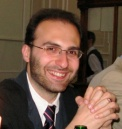### Comprehensive List of Researchers "Information Knowledge"

Department of Computer Science and Mathematical Informatics

Name
BUSCEMI,Francesco
Group
Mathematical Modeling and Analysis Group
Title
Professor
Degree
Ph. D. (Philosophy)
Research Field
Quantum Foundations / Quantum Measurements / Quantum Communication / Quantum Computing#### Current Research

##### Study of fundamental principles in quantum information theory
RESEARCH OUTLINE

Processing information always amounts to manipulating physical systems, and viceversa. Hence, the ultimate limits to information processing are posed by the very laws of physics. Quantum Information Theory is an interdisciplinary, rapidly expanding field of research devoted to the study of information processing, in the case in which the underlying physical systems obey the rules of quantum theory.

My research interests cover fundamental aspects in quantum theory (quantum measurement processes, information-disturbance tradeoff, quantum decoherence, quantum entanglement), quantum information theory (single-shot and asymptotic coding theorems for general quantum resources), quantum statistics (quantum games, comparison of quantum statistical models). The techniques I use are mainly borrowed from linear algebra, group theory, convex analysis, statistics, and information theory.

Quantum Measurement Theory

The basis of any information processing task relies on the notion of information extraction, or read-out. The mathematical structure needed to describe such a task in full generality, as showed by M. Ozawa, is provided by the notion of quantum instruments. Any noisy process, evolution, encoding and decoding operation can be conveniently modeled as a specific quantum instrument. This is the reason why the study of quantum instruments and quantum measurement processes enjoys a privileged position within the field of quantum information theory. Within this area, I'm especially interested in characterizing quantum instruments according to the disturbance they cause on the measured object. In fact, intuitively speaking, for a fixed amount of information extracted, some quantum instruments cause less disturbance than others. The least disturbing quantum instruments therefore constitute, in a sense, a class of "optimal" measurement processes. This research topic has implications also in the emerging field of quantum technologies, where the design of accurate, though delicate, measuring apparatuses is of crucial importance.

Quantum Statistics

The task in which an experimenter tries to learn about the true value of an unknown parameter by observing a random variable whose distribution depends on such a value, is generally called a statistical estimation task or a statistical decision problem. The mathematical structures needed to describe such a situation in classical statistics are called statistical models, i.e. families of probability distributions (or, more generally, measures) indexed by a parameter set, which represents the unknown parameter one wants to learn about in the estimation process. An important subject in classical statistics, with implications also in the field of the statistical design of experiments, is the comparison of statistical models in terms of their information value in statistical decision problems. At present, I'm actively working to extend the concepts of statistical model comparison to the quantum setting, where probability measures are replaced by density operators acting on an Hilbert space.

Quantum Information Theory

Since after the work of Claude Shannon, information theory can be considered as that body of mathematical literature characterizing optimal rates at which various information-processing tasks (e.g. source coding, noisy channel coding, etc) can be achieved. Usually, such rates are computed in the limit of asymptotically many instances of the same information theoretical setting, thought of as identically and independently repeated an infinite number of times. One of the biggest successes of quantum information theory has been to elegantly generalize many of these theorems to the case in which the underlying algebra is non-commutative. This field of research is usually called Quantum Shannon Theory. However, in order to assess the performance of real-world information processing tasks, it is important to characterize them when the unphysical assumptions of identical, independent, and infinite repetitions are removed from the theory. In classical information theory, new tools were developed to deal with such a generalized scenario, i.e. the Information Spectrum Approach and the Single-Shot Approach. Recently, these tools have been extended also to quantum information theory. Part of my research is devoted to the study of such advanced tools and their application to characterize quantum information-theoretical protocols, especially in relation with the theory of quantum entanglement and quantum communication.

#### Career

• Francesco Buscemi received the PhD in Theoretical Physics from the University of Pavia in 2006. From 2006 to 2008 he was Researcher at the Quantum Computation and Information Project, JST, Tokyo. From 2008 to 2009 he was Research Associate at the Statistical Laboratory of the Department of Pure Mathematics and Mathematical Statistics, University of Cambridge, UK. From 2009 to 2014, Francesco Buscemi has been a Tenure-Track Associate Professor at the Institute for Advanced Research of Nagoya University. From April 2014, he is Tenured Associate Professor in the Group of Mathematical Modeling and Analysis at the Graduate School of Information Science of Nagoya University.